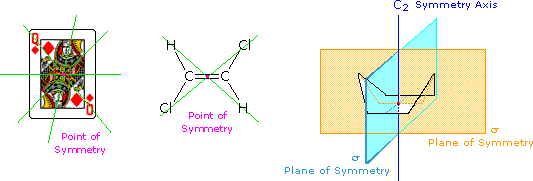# Chirality and Symmetry

$$\newcommand{\vecs}{\overset { \rightharpoonup} {\mathbf{#1}} }$$ $$\newcommand{\vecd}{\overset{-\!-\!\rightharpoonup}{\vphantom{a}\smash {#1}}}$$$$\newcommand{\id}{\mathrm{id}}$$ $$\newcommand{\Span}{\mathrm{span}}$$ $$\newcommand{\kernel}{\mathrm{null}\,}$$ $$\newcommand{\range}{\mathrm{range}\,}$$ $$\newcommand{\RealPart}{\mathrm{Re}}$$ $$\newcommand{\ImaginaryPart}{\mathrm{Im}}$$ $$\newcommand{\Argument}{\mathrm{Arg}}$$ $$\newcommand{\norm}{\| #1 \|}$$ $$\newcommand{\inner}{\langle #1, #2 \rangle}$$ $$\newcommand{\Span}{\mathrm{span}}$$ $$\newcommand{\id}{\mathrm{id}}$$ $$\newcommand{\Span}{\mathrm{span}}$$ $$\newcommand{\kernel}{\mathrm{null}\,}$$ $$\newcommand{\range}{\mathrm{range}\,}$$ $$\newcommand{\RealPart}{\mathrm{Re}}$$ $$\newcommand{\ImaginaryPart}{\mathrm{Im}}$$ $$\newcommand{\Argument}{\mathrm{Arg}}$$ $$\newcommand{\norm}{\| #1 \|}$$ $$\newcommand{\inner}{\langle #1, #2 \rangle}$$ $$\newcommand{\Span}{\mathrm{span}}$$$$\newcommand{\AA}{\unicode[.8,0]{x212B}}$$

All objects may be classified with respect to a property we call chirality (from the Greek cheir meaning hand). A chiral object is not identical in all respects (i.e. superimposable) with its mirror image. An achiral object is identical with (superimposable on) its mirror image. Chiral objects have a "handedness", for example, golf clubs, scissors, shoes and a corkscrew. Thus, one can buy right or left-handed golf clubs and scissors. Likewise, gloves and shoes come in pairs, a right and a left. Achiral objects do not have a handedness, for example, a baseball bat (no writing or logos on it), a plain round ball, a pencil, a T-shirt and a nail. The chirality of an object is related to its symmetry, and to this end it is useful to recognize certain symmetry elements that may be associated with a given object. A symmetry element is a plane, a line or a point in or through an object, about which a rotation or reflection leaves the object in an orientation indistinguishable from the original. Some examples of symmetry elements are shown below.The face playing card provides an example of a center or point of symmetry. Starting from such a point, a line drawn in any direction encounters the same structural features as the opposite (180º) line. Four random lines of this kind are shown in green. An example of a molecular configuration having a point of symmetry is (E)-1,2-dichloroethene. Another way of describing a point of symmetry is to note that any point in the object is reproduced by reflection through the center onto the other side. In these two cases the point of symmetry is colored magenta.

The boat conformation of cyclohexane shows an axis of symmetry (labeled C2 here) and two intersecting planes of symmetry (labeled σ). The notation for a symmetry axis is Cn, where n is an integer chosen so that rotation about the axis by 360/nº returns the object to a position indistinguishable from where it started. In this case the rotation is by 180º, so n=2. A plane of symmetry divides the object in such a way that the points on one side of the plane are equivalent to the points on the other side by reflection through the plane. In addition to the point of symmetry noted earlier, (E)-1,2-dichloroethene also has a plane of symmetry (the plane defined by the six atoms), and a C2 axis, passing through the center perpendicular to the plane. The existence of a reflective symmetry element (a point or plane of symmetry) is sufficient to assure that the object having that element is achiral. Chiral objects, therefore, do not have any reflective symmetry elements, but may have rotational symmetry axes, since these elements do not require reflection to operate. In addition to the chiral vs achiral distinction, there are two other terms often used to refer to the symmetry of an object. These are:

1. Dissymmetry: The absence of reflective symmetry elements. All dissymmetric objects are chiral.
2. Asymmetry: The absence of all symmetry elements. All asymmetric objects are chiral.

Models of some additional three-dimensional examples are provided on the interactive symmetry page. The symmetry elements of a structure provide insight concerning the structural equivalence or nonequivalence of similar component atoms or groups Examples of this symmetry analysis may be viewed by Clicking Here.